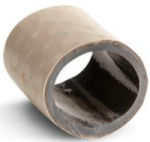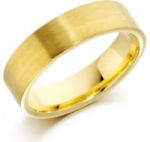Search IntMath
Close

# 4b. Shell Method: Volume of Solid of Revolution

by M. Bourne

In the last section we learned how to use the Disk Method to find the volume of a solid of revolution. In some cases, the integral is a lot easier to set up using an alternative method, called Shell Method, otherwise known as the Cylinder or Cylindrical Shell method.

## a. Shell Method formula

The formula for finding the volume of a solid of revolution using Shell Method is given by:

V = 2pi int_a^b rf(r)dr

where r is the radius from the center of rotation for a "typical" shell.

We'll derive this formula a bit later, but first, let's start with some reminders.

Continues below

## b. Volume of a Hollow Cylinder

Examples of hollow cylinders:Hose pipeWedding ring

The volume, V of the material needed to make such hollow cylinders is given by the following, where R is the radius of the outer wall of the cylinder, and r is the radius of the inner wall:

V = "outer volume" - "hole volume"

= pi R^2 h - pi r^2 h

= pi h (R^2 - r^2)

Another way to go about it (which we use in this section) would be to cut the cylinder vertically and lay it out flat. The width of the flat piece would be 2pir, the circumference of the cylinder, and the thickness is (R-r). The volume would be given by approximately:

V = 2pir xx h xx (R-r)

### Example 1: Wedding ring

A wedding ring has outer radius 10 mm and inner radius 8 mm. Its height (when laying flat on a table) is 3 mm. Find the volume using both methods mentioned above.

The volume is given by:

(a) Difference method

V = pi h (R^2 - r^2)

= pi (3) (10^2 - 8^2)

=108pi

= 339.3\ "mm"^3

(b) Laying out flat

V ~~ 2pi r xxh xx (R-r)

= 2 pi (10)(3)(2)

=120pi

= 377.0\ "mm"^3

The second one is an approximation, but provides a useful way of calculating volumes of solids of revolution.

Let's now see how to find the volume for more unusual shapes, using the Shell Method.

## i. Rotation around the y-axis

### Example 2: Cone

Consider rotating the triangle bounded by y=-3x+3 and the two axes, around the y-axis. It forms a cone.

Area under the curve y=-3x+3 from x=0 to x=1 rotated around the y-axis.

We can think of a solid of revolution as the sum of a set of hollow cylinders. We first consider the simple case of a cone, so that we can easily check our calculations.

We use a set of cookie cutters (and some imagination) to slice the cylinder into a set of hollow cylinders, as follows.

Cone showing typical shells.

Here are the individual shells for the above example. The 5th one is actually a solid cylinder.

Typical shells that approximate our cone.

As an example, we take one of the shells, cut it vertically, and lay it out flat.

Typical shell cut vertically and rolled out flat.

The volume of the above thin box shape is lxxwxxh.

The length is given by 2pir (this is just the circumference of the shell, and r is the radius of the shell). The height is given by the function value for the particular shell, f(r), and the width is the thickness of the shell, which we write as Deltar (that is, "change in r".)

So the volume is:

V = 2pi rxx f(r) xx Delta r

#### Approximate Volume

Our 5 shells in the cone example are each 0.2 units thick. The height of each one is given by their appropriate function values, as follows:

f(0.1) = - 3(0.1) + 3 = 2.7

f(0.2) = - 3(0.3) + 3 = 2.1

f(0.4) = - 3(0.5) + 3 = 1.5

f(0.6) = - 3(0.7) + 3 = 0.9

f(0.8) = -3(0.9) + 3 = 0.3

So the total volume given by our five shells is:

"sum" = 2pi[(0.1)(2.7)(0.2)  + (0.3)(2.1)(0.2)  + (0.5)(1.5)(0.2) + (0.7)(0.9)(0.2) {: + (0.9)(0.3)(0.2)]

=2pi(0.51)

~~3.204

Check: We know that the exact volume of a cone is given by V=(pir^2h)/3. So in this case, the volume will be:

V = (pi(1)^2(3))/3 = pi ~~ 3.1416

Our approximation is pretty good.

If we slice the cone such that there are many very thin shells, our value for the volume will get even closer to the real value.

In general, we have:

V= sum_a^b 2pi rf(r)Delta r

In the case of our cone, and rotation around the y-axis, the radius is actually x (the distance from the center of rotation to the shell) and the height of each cylindrical shell is given by f(x), which in this case is f(x)=-3x+3. So we have:

V= sum_0^1 2pi x(-3x+3)Delta x

As we take more and more thinner slices, Deltax gets very small, and as it tends to 0, our volume is given by the integral:

V = int_0^1 2pi(x(-3x+3)dx

=2pi int_0^1 (-3x^2 + 3x)dx

=2pi [-x^3 +(3x^2)/2]_0^1

= pi

## General Formula for the Volume

Earlier, we saw this formula for the volume:

V=2pi int_a^b rf(r)dr

The r changes depending on whether we are rotating around the x- or y-axis. In the above case, we are rotating about the y-axis, so r is x (since the radius increases along the x-axis), and the height of each shell is given by f(x).

So when rotating around the y-axis, we write it as:

V=2pi int_a^b x f(x)dx

The x represents the radius of a "typical" shell, and f(x) gives us the height of that typical shell.

### Example 3: Cubic function

Find the volume of the solid formed by rotating the area bounded by y=x^3+x^2+1, the x- and y-axes, and the line x=2 around the y-axis.

The area between y=x^3+x^2+1, the axes and x=2 rotated round the y-axis.

Applying the formula from above, for rotation around the y-axis, 2pi int_a^b xf(x)dx, we have:

V = 2pi int_0^2 (x(x^3+x^2+1)dx

=2pi int_0^1 (x^4 + x^3 + x)dx

=2pi [(x^5)/5 +(x^4)/4 + (x^2)/2]_0^2

= (124pi)/5

### Challenge

Can you do the above question using disk method? I suspect not.

One problem with disk method is we have to be able to express the given function in terms of y. It is very difficult to solve a cubic equation, and we are stumped. In this case, shell method is much easier.

## ii Rotation around the x-axis

### Example 4: Rugby football

A regulation rugby football is approximately 30 cm long and 54 cm in circumference at its widest point. It is a prolate spheroid (an elongated ellipsoid), with major axis 30 cm and the minor axis 17 cm.

Find its volume. (We did a similar problem in Exercise 4 of the previous page, Volume by Disk Method, but in that case we rotated around the y-axis.)

Here's the equation of the ellipse representing the cross-section of our football. (See background on this at: Ellipses.)

x^2/15^2 + y^2/8.5^2 = 1

Since it is symmetric, we'll take the right half of this ellipse and rotate it around the x-axis, as follows. (We'll double our answer for the complete volume at the end.)

The graph of the top right half of x^2/15^2 + y^2/8.5^2 = 1, rotated around the x-axis.

The graph also shows a typical shell. We can see the radius of the shell depends on the distance up the y-axis and the "height" of the shell will be given by the inverse function g(y).

V= int_c^d 2pi yg(y)dy

The lower and upper limits of the integral and the upper and lower y-values for the function.

Expressing x^2/15^2 + y^2/8.5^2 = 1 in terms of y (that is, solving for x), we have:

g(y) = 15sqrt(1-y^2/8.5^2)

Substituting that expression in our formula, and integrating with respect to y, we have:

V= 2piint_0^8.5 y xx 15sqrt(1-y^2/8.5^2)dy = 30pi int_0^8.5 y sqrt(1-y^2/8.5^2)dy

We need to use a substitution, u = 1-y^2/8.5^2, giving du = -(2y)/8.5^2 dy, which we change into the form -8.5^2/2du = y dy. (See more on Substitution in Integration.)

As y goes from 0 to 8.5, u goes from 1 to 0.

The integral becomes:

30pi int_1^0 -8.5^2/2 sqrt(u)du

= -15(8.5^2)pi int_1^0 sqrt(u)du

= -15(8.5^2)pi [2/3 u^(3//2)]_1^0

= 10(8.5^2)pi

~~ 2323.5\ "cm"^3

So the volume for a rugby football is approximately 2xx2323.5 = 4647\ "cm"^3.

## d. When to use the Disk versus Shell Mathod

It's essential to sketch the curve and the rotated object, and to include the "typical" disk (or "typical shell"). It makes it much easier to see what is happening and how best to proceed.

If the question doesn't specify which method to use, generally the disk method is easier to set up.

However, as we learned above, if we rotate a cubic (or higher power of x) around the y axis, we can't use disk (or washer) method, since it's hard (or impossible) to re-express the function in terms of y only. In this case, we need to use Shell Method.

## Problem SolverThis tool combines the power of mathematical computation engine that excels at solving mathematical formulas with the power of GPT large language models to parse and generate natural language. This creates math problem solver thats more accurate than ChatGPT, more flexible than a calculator, and faster answers than a human tutor. Learn More.